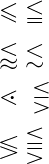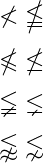# How to write less than(<) symbol in LaTeX?

Less than is the inequality mathematical symbol that is on your keyboard. And in latex, you can use the direct less than symbol as a command to represent this symbol.

Symbol Less than symbol
Unicode/type U+2032 / Inequality
Package Default
Command <
Example a &lt; b → a < b
\documentclass{article}
\begin{document}
% You cannot directly use this symbol in text mode
$a < b$
$p < q$
\end{document}

Output :

a < b
p < q


This symbol is combined with other different symbols to create new inequality symbols that are not on your keyboard.

So, for this, you need to take the help of external commands or packages. For example

\documentclass{article}
\usepackage{amssymb}
\begin{document}
$\leqslant \; \leqq$
$\lessapprox \; \lesssim$
$\lessdot \; \lesseqgtr$
$\lessgtr \; \lesseqqgtr$
\end{document}

Output :And you can create a not less than type symbol by using the not symbol with each of the above symbols.

\documentclass{article}
\usepackage{amssymb}
\begin{document}
$\nless \; \nleqq$
$\nleqslant \; \nleq$
$\lneqq \; \lneq$
$\lnapprox \; \lnsim$
\end{document}

Output :Many of you will think that it is better to have double less than symbol from the keyboard sitting side by side for much less than symbol. But no, the best practice is to use commands.

\documentclass{article}
\usepackage{txfonts}
\begin{document}
$a << b$
$p << q$
% below latex command are best practice for much less than symbol
$a \ll b$
% \nll command from txfonts package
$p \nll q$
\end{document}

Output :In this tutorial, all the less than related symbols are denoted with the help of LaTeX. Of course, you have found the answer to your question.#### Md Jidan Mondal

LaTeX expert with over 10 years of experience in document preparation and typesetting. Specializes in creating professional documents, reports, and presentations using LaTeX.